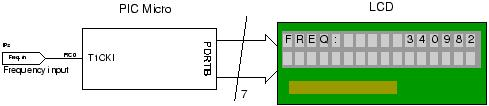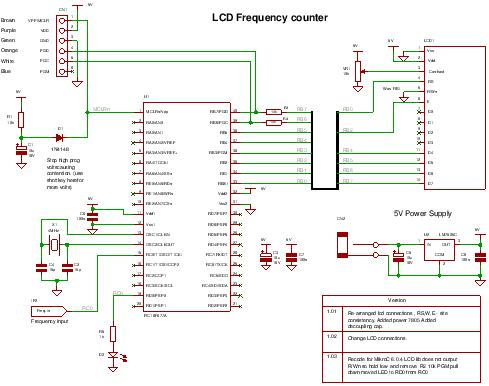# A PIC frequency counter operating up to about 50 MHz. usnig pic microcontoller

This PIC frequency counter project uses an LCD to display the frequency and PIC timer 1 to measure the input signal and Timer0 to measure the timing period.

It uses Timer 1 in 16 bit counter mode to count the input signal edges and overflows of the counter are accumulated to give the total count in multiples of 65536.Adding the current value of the counter at the end gives the total count.The crystal oscillator is chosen to be 4MHz (Fosc) so that the processor internal clock is 1MHz (Fosc/4). All you do is count 1e6 processor clocks (Timer0) to give a 1second count period.

Since the measurement time is 1 second the final count is actually the frequency of the input signal.

#### Specification

 Min frequency 1Hz Max frequency ~50MHz (limited by input pin characteristics).(Tested using TTL oscillator at 20MHz). Input signal level TTL

Note: The exact maximum operating frequency is determined by the PIC input pin characteristic.

For the general theory of operation of this circuit and notes on frequency counting of this pic frequency counter click here.

## Pic frequency counter Hardware

The hardware is simple and the main blocks are shown in the diagram below.

The LCD is used in 4 bit mode interface so you only need 4 data lines and three control lines and it then fits into a single 8 bit port.

The crystal oscillator is simply a crystal and two capacitors connected to the PIC oscillator port at OSC1 and OSC2. The capacitors can both be fixed at the same value unless you want to tune it using a frequency reference. If you don’t have an accurate reference then use fixed capacitors.The PIC micro can be any type that has Timer 0 and Timer 1 hardware modules and and has enough memory to hold the program ~1.6k words.

The LED is toggled to indicate that the processor is alive – so if there is no input signal you can tell that the software is working. Also if there is no input signal the the LCD displays a flashing zero.

You can program the PIC in circuit through the ICSP connector in circuit.

## Description

To time a 1 second count Timer0 is used. Since the main clock is running at 4MHz then the processor clock (Fosc/4) is 1MHz which is the rate that Timer0 is set-up to use i.e. the internal clock. Therefore we need to get a 1 second count using that timer. Since the timer is only 8 bits long you can use the fact that an interrupt is generated when it overflows – you can then count these overflows to get near to 1e6 counts.

Since the overflow occurs every time that the counter passes 256 we need to count 1e6/256 overflows

For more detail: A PIC frequency counter operating up to about 50 MHz.